# Affine coordinate system

A rectilinear coordinate system in an affine space. An affine coordinate system on a plane is defined by an ordered pair of non-collinear vectorsand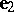(an affine basis) and a point(the coordinate origin). The straight lines passing through the point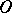and parallel to the basis vectors are known as the coordinate axes. The vectors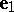anddefine the positive direction on the coordinate axes. The axis parallel to the vectoris called the abscissa (axis), while that parallel to the vectoris called the ordinate (axis). The affine coordinates of a pointare given by an ordered pair of numberswhich are the coefficients of the decomposition of the vector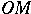by the basis vectors: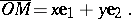The first numberis called the abscissa, while the second number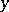is called the ordinate of.
An affine coordinate system in three-dimensional space is defined as an ordered triplet of linearly-independent vectors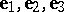and a point. As in the case of the plane, one defines the coordinate axes — abscissa, ordinate and applicate — and the coordinates of a point. Planes passing through pairs of coordinate axes are known as coordinate planes.